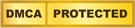# Simulating Cloth for 3D Games

Published Date
27 - Apr - 2017
| Last Updated
27 - Apr - 2017Introduction

We all live in the real world where things behave according to the laws of physics that we learned about in high school or college. Because of this, we're all expert critics about what looks right or more often wrong in many 3D games. We complain when a character's feet slide across the ground or when we can pick out the repeating pattern in the animation of a flag blowing in the wind. Adding realistic physical simulation to a game to improve these effects can be a giant effort and the rewards for the time invested haven't proven to be worthwhile, yet.

Often, though, it's possible to incrementally add elements to a game that can provide increased realism without extremely high risks. Improving the animation behavior of simple cloth objects like flags in the wind and billowing sails is one area where realism increases without the 18 month development risk of introducing a full-fledged physics engine. Not that I don't want to see more games with all-out physics happening, but I think there are some simple things that can be done with cloth objects in the meantime to improve realism and save modelers time.

At the Game Developers Conference in March 2000, I presented my implementation of two techniques for simulating cloth. I was pointed to another, more recent, technique by someone who attended the class. In this paper I'll recap what I presented about at the conference and include information about the newer technique. Hopefully you'll be able to take the ideas I present here and add some level of support for cloth simulation into your title.

2. Background

Various researchers have come up with different techniques for simulating cloth and other deformable surfaces. The technique that is used by all three methods presented here, and by far the most common, is the idea of a mass-spring system. Simply put, a continuous cloth surface is discretized into a finite number of particles much like a sphere is divided into a group of vertices and triangles for drawing with 3D hardware. The particles are then connected in an orderly fashion with springs. Each particle is connected with springs to its four neighbors along both the horizontal and vertical axes. These springs are called "stretch'" springs because they prevent the cloth from stretching too much. Additional springs are added from each particle to its four neighbors along the diagonal directions. These "shear" springs resist any shearing movement of the cloth. Finally, each spring is connected to the four neighbors along both the horizontal and vertical axes but skipping over the closest particles. These springs are called "bend" springs and prevent the cloth from folding in on itself too easily.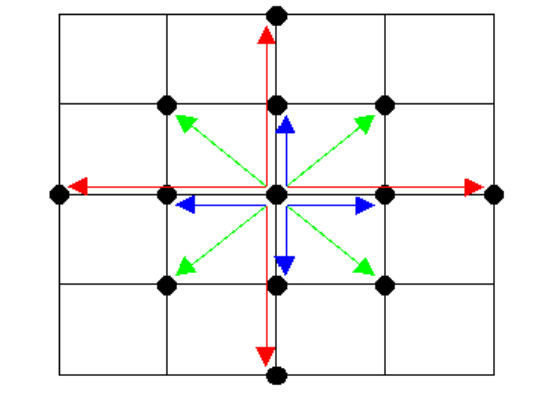Figure 1 - Stretch (blue), Shear (green), and Bend (red) springs

Figure 1 shows a representation of a mass-spring system using the previously mentioned stretch, shear, and bend springs. When rendering this surface, the masses and springs themselves are not typically drawn but are used to generate triangle vertices. The nature of the cloth simulation problem involves solving for the positions of the particles at each frame of a simulation. The positions are affected by the springs keeping the particles together as well as by external forces acting on the particles like gravity, wind, or forces due to collisions with other objects or the cloth with itself.

In the next section we'll look at the problem that we're trying to solve to realistically animate a cloth patch. Much of this will be very familiar to anyone who has already experimented with cloth simulation. Feel free to skip to Section 4 if you just want details on the various implementations I tried.

The Cloth Problem

Like any other physical simulation problem, we ultimately want to find new positions and velocities for objects (cloth particles in our case) using Newton's classic law:  or more directly . This says that we can find the acceleration () on a particle by taking the total force () acting on the particle and dividing by the mass (m) of the particle. Using Newton's laws of motion, we can solve the differential equations and to find the velocity () and position () of the particle. For simple forces, it may be possible to analytically solve these equations, but realistically, we'll need to do numerical integration of the acceleration to find new velocities and integrate those to find the new positions.

In Sections 3.1 through 3.3 we'll take a high level look at explicit integration, implicit integration, and adding post-integration deformation constraints for solving the equations of motion for cloth particles. Many excellent in-depth articles have been written about various aspects of physics simulation including cloth simulation. I'd highly recommend the articles by Jeff Landeri, ii, and Chris Heckeriii if you haven't already read them.

3.1. Explicit Integration

One of the simplest ways to numerically integrate the differential equations of motion is to use the tried-and-true method known as Euler's method. For a given initial position, , and velocity, , at time and a time step, , we can calculate a new position, , and velocity, , using a Taylor series expansion of the above differential equations and then dropping some terms (which may introduce error, ):Unfortunately, Euler's method takes no notice of quickly changing derivatives and so does not work very well for the stiff differential equations that result from the strong springs connecting cloth particles. Provotivintroduced one method to overcome this problem and Desbrunv later expanded on this. We'll examine these in more depth in Section 3.3. Until then, let's look at implicit integration.

3.2. Implicit Integration

Given the problem with Euler's method for stiff differential equations and knowing that the problem still exists for other similar "explicit" integration methods, some researchers have worked with what are known as "implicit" integration methods. Baraff and Witkinvi presented a thorough examination of using implicit integration methods for the cloth problem. Implicit integration sets up a system of equations and then solves for a solution such that the derivatives are consistent both at the beginning and the end of the time step. In essence, rather than looking at the acceleration at the beginning of the time step, it finds an acceleration at the end of the time step that would point back to the initial position and velocity.

The formulation I'm using here is from the Baraff and Witkin paper except I've used  to represent the position of the particles rather than . The system of equations isHere M-1 is the inverse of a matrix with the mass of the individual particles along the diagonal. If all the particles are the same mass, we can just divide by the scalar mass, m. Like was done in the explicit case, we use a Taylor series expansion of the differential equations to form the approximating discrete system:The top row of this system is trivial to find once we've found the bottom row, so by plugging the top row into the bottom row, we get the linear system:3.3. Deformation Constraints

When using either explicit integration or implicit integration to determine new positions and velocities for the cloth particles, it is possible to further improve upon the solution using deformation constraints after the integration process. Provot proposed this method in his paper and Desbrun further combined this with a partial implicit integration technique to achieve good performance with large time steps.

The technique is very simple and easy to implement. Once an integration of positions and velocities has been done, a correction is applied iteratively. The correction is formed by assuming that the particles moved in the correct direction but that they may have moved too far. Particles are then pulled together along the correct direction until they are within the limits of the deformation constraints. The process can be applied multiple times until convergence is reached within some tolerance or there is no time left for the process to be able to maintain a given frame rate. Using deformation constraints can take a normally unstable system and stabilize it quite well. I've found that using a fixed number of iterations typically works well.

Now that we've taken a brief look at integration techniques and how to improve upon the results, let's have a look at the implementations I did. The source code for my implementations can be downloaded and used in your application or just examined for ideas.

Implementation

I tried implementing a simple cloth patch using three techniques: explicit integration with deformation constraints, implicit integration, and semi-implicit integration with deformation constraints. The sample application depicted in Figure 2 shows a simple cloth patch that can be suspended by any or all of its four corners.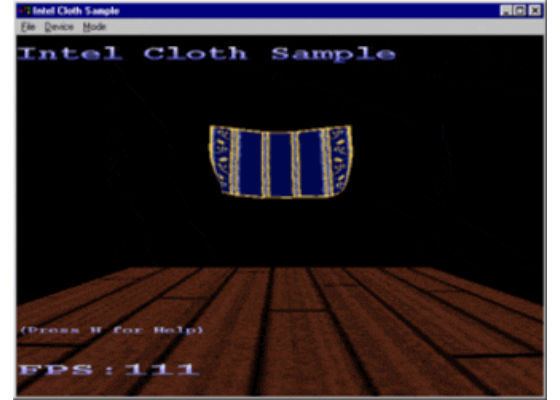Figure 2 - Cloth Sample Application

Gravity pulls downward on the particles and stretch, shear, and bend springs keep the particles together as a cloth patch. A wireframe version of the cloth is shown in Figure 3. Two triangles are produced for every four particles forming a grid square.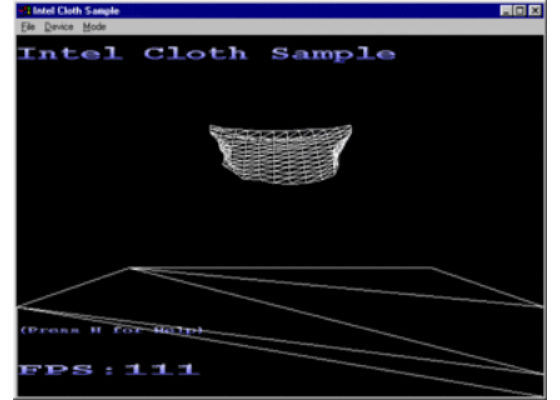Figure 3 - Wireframe view of cloth patch

I'll discuss the implementation specifics here with a simple analysis of the results in Section 5.

4.1. Basics

For the three implementations, I shared a lot of code. Everything is written in C++ with a rough attempt at modularizing the cloth specific code into a set of physics/cloth related classes. I used a 3D application wizard to create the framework and then added the cloth specific stuff. Information about the 3D AppWizard, for those interested, can be found in the article Creating A Custom Appwizard for 3D Development.

When wading through the source code, you'll find that there are quite a few files. Most of the files that pertain to the cloth simulation are in the files that begin with "Physics_". In addition to these I also created a "ClothObject" class with corresponding filenames which is instantiated and manipulated from the "ClothSample" class.

I experimented with performance with both single-precision and double-precision floating point numbers. To easily change this, I created a typedef in Physics.h for a "Physics_t" type that is used anywhere you would normally use "float" or "double". I found (expectedly) that performance slowed when using double-precision numbers and I didn't notice any improved stability. Your mileage may vary especially if you add support for collision detection and response.

Mass-Spring System

The mass-spring system is implemented as a particle system. This basically means that I don't do any handling of torque or moments of inertia. Within the Physics_ParticleSystem class, I allocate necessary information for the various integration schemes and I allocate large vectors for holding the positions, velocities, forces, etc. of the individual particles. I maintain a linked list of forces that act on the particles.

With this implementation there's no way of dynamically changing the number of particles in the system (although forces can be added and removed). For the implicit integration scheme, I allocate some sparse, symmetric matrices to hold the derivatives of the forces and temporary results. For the semi-implicit scheme, I allocate some dense, symmetric matrices to hold the Hessian matrix and inverse matrix, W, for filtering the linear component of the forces.

Regardless of which integration scheme is used we'll use the same overall update algorithm. Pseudo-code for updating the cloth is shown in Figure 4. This routine, Update, is called once per frame and in my implementation uses a fixed time step. Ideally, you'll want to use a variable time step. Remember that doing so can have an impact on performance, especially in the semi-implicit implementation of Desbrun's algorithm because a matrix inversion would be done at each frame where the step size changed. Clearing the accumulators is a no-brainer so I'll just dive into the other three steps of the algorithm in further detail.

4.2.1. Calculating forces and derivatives

My implementation only has two types of forces, a spring force and a gravity force. Both are derived from aPhysics_Force base class. During the update routine of the particle system, each force is enumerated and told to apply itself to the fo rce and force derivative accumulators. Force derivatives are only needed when
using the implicit integration scheme (actually, they're needed for the semi-implicit integration scheme, but are handled differently).

The gravity force is simple and just adds a constant (the direction and magnitude of gravity: 0,-9.8,0 in my case) to the "external" force accumulator. I maintain separate "internal" and "external" accumulators to support the split integration scheme proposed by Desbrun. The downside to this is that I would really need a separate spring force for handling user supplied force to the cloth because the spring force as implemented assumes that it is acting internally to the cloth only.

The spring force is a simple, linear spring with damping. I derived the force from a condition function as was done in the Baraff/Witkin paper. Unlike the Baraff/Witkin paper's use of separate condition functions for stretching, shearing and bending on a per triangle basis, I use just one condition function for a linear spring connecting two particles. The condition function I used was where p0 and p1 are the two particles affected by the spring and dist is the rest distance of the spring. Forces were calculated as derivatives of the energy function formed by the condition function: .

The Desbrun paper uses the time step and spring constant to apply damping but I apply damping as derived by the Baraff/Witkin paper. The damping constant I use is a small multiple of the spring constant.

4.2.2. Integrating forces and updating positions and velocities

By far, the trickiest code to understand is that for integrating the forces to determine new velocities and positions for the cloth particles. We'll start with the simplest case, the explicit integration scheme with deformation constraints.

4.2.2.1. Explicit integration with deformation constraints

Using explicit Euler integration is a straightforward application of equations. The acceleration is found by dividing the force for each particle by the particles mass (actually, we store 1/mass and then do a multiplication). Then, the acceleration is multiplied by the time step to update the velocities. The new velocities are multiplied by the time step to update the positions. The new positions are actually stored in a temporary location so that the deformation constraints can be applied. To apply the deformation constraints, each spring force is asked to "fixup" its associated particles. Basically, if the length of the spring has exceeded a maximum value (determined as a multiple of the rest length of the spring), then the particles are pulled closer together. Finally, we take the fixed-up temporary positions, subtract the starting positions and divide by the time step to get the actual velocities needed to achieve the end state. Then we copy the temporary positions to the actual positions vector and we're ready to render.

4.2.2.2. Implicit integration

At the other end of the spectrum in terms of difficulty is doing full implicit integration using equation (1.6). For this, we form a large, linear system of equations and then use an iterative solution method called the pre-conditioned conjugate gradient method. The Baraff/Witkin paper goes into details on this and explains the use of a filtering process for constraining particles. In my implementation, I inlined the filtering function everywhere it was used. I won't go into the ugly details of the conjugate gradient method, but I will explain briefly some of the tricks I used to improve performance. For one, the large sparse matrices that get formed  are all symmetric, so I cut storage requirements almost in half by only storing the upper triangle of the matrices. In doing so, I had to think carefully about the matrix-vector multiply routines. Secondly, in cases where we would actually be using a matrix but one that only had non-zero elements along the diagonal, I just stored the matrix as a vector. I added some specialized routines to the Physics_LargeVector class for "inverting" the vector which just replaced each element with one over the element. Finally, I didn't do any dynamic allocation of the temporary sparse matrices because the overhead would have been too severe. So I ended up keeping some temporary matrices as private members of the Physics_ParticleSystem class.

4.2.2.3. Semi-implicit integration with deformation constraints

The last integration method I tried was a semi-implicit method as described by Desbrun. Desbrun divided the internal forces acting on the cloth into linear components and non-linear components. The linear components could then be easily integrated using implicit integration without having to solve a linear system. Instead, a large constant matrix is inverted once and then just a matrix multiply is required to do the integration. The non-linear components are approximated as torque changes on a global scale when using his technique. In addition, deformation constraints are used to prevent overly large stretching. As mentioned previously, I created a Physics_SymmetricMatrix class for storing the Hessian matrix of the linear portion of the internal cloth forces. The Hessian matrix is used in place of from equation (1.6) and because of the linear nature imposed by Desbrun's splitting of the forces, is zero. Due to the splitting of the problem into a linear and non-linear portion, we don't need to solve a linear system as we did in the Baraff/Witkin implementation. Rather, we can just "filter" the internal forces by multiplying by the inverse matrix where I is the identity matrix, dt is the time step, m is the mass of a particle, and H is the  Hessian matrix. We then need to compensate for errors in torque introduced by the splitting. I'd refer the reader to the Desbrun article for more information about the technique. As in the explicit integration scheme, once we've integrated the forces and obtained new velocities and positions (again stored in a temporary vector) we can apply the deformation constraints. See above for details.

Extra Tidbits

While the above explanations of the update loops give the core information about how the cloth patch animates, there is some secondary information that is useful to know when looking through the code. I'll go through several different areas and unless otherwise noted, the text refers to all three update methodologies.

Each particle in the mesh can belong to at most six triangles. I generate a normal for each triangle and then add these and normalize to get the normal at each particle. This process doesn't seem to consume much time, but if every processor cycle is critical, you can choose to average less than six normals.

For the semi-implicit implemenation, I need to form the Hessian matrix that corresponds to the way the particles are connected by the springs. I do this once, upfront, because the spring constants don't change and so the Hessian matrix doesn't change. For each spring, it's Prepare Matrices method is called. This method sets the appropriate elements in the Hessian matrix that the spring affects. Prepare Matrices also is called to "touch" elements of the sparse matrices that will be used by the implicit implementation. This enables the memory allocation to happen only once.

I incorporated a very simplistic collision detection for the cloth with the ground plane. If you use the number keys (0,1,2,3) to toggle constraints on the corners, you can get the cloth to move downward. When it hits the floor, I stop all movement in the downward direction and fix the particles to the plane of the floor. There's no friction, so it's not very realistic. For the implicit implementation, I imposed constraints and particle adjustments as describe by Baraff and Witkin, however things tend to jump unstably as the cloth hits the floor. It's possible a smaller time step is needed but I didn't investigate further.

Both the explicit and semi-implicit routines use particles with infinite mass to constrain them. Because of this, the Fixup routine for applying the deformation constraints looks at the inverse mass of each particle and only moves the particle if its mass is non-infinite (which means the inverse mass is non-zero).

While running the demo the following keys affect the behavior of the cloth:

• P - Pauses the animation of the cloth
• W - Toggles wireframe so you can see the triangles
• X - Exits the demo
• F - Toggles to fullscreen mode
• H - Brings up a help menu showing these keys
• R - Resets the cloth to its initial position - horizontal to the floor and a bit above it
• 0, 1, 2, 3 - Toggles constraints for the four corners of the cloth

Finally, the configuration of the cloth simulation (number of particles, strength of springs, time step, etc.) is contained in Cloth.ini. I added comments for each entry in the file so look there if you want to play around with things. By default the integration method is explicit.

Which Method is Best?

Since I've covered three different techniques for updating the cloth, I'm sure you're wondering what the best m ethod is. Well, for the case I tried the explicit implementation is clearly the fastest as the results in Figure 5 show. This table was generated from running the sample code on an Intel® Pentium® III processor-based system running at 600 Mhz with Microsoft Windows* 98 and DirectX* 7.0. The graphics card was a Matrox* G-400 with the resolution set to 1024x768 @ 60Hz and the color depth set to 16-bit. I used a fixed time step of 0.02 seconds which would be appropriate for a frame rate of 50 frames per second.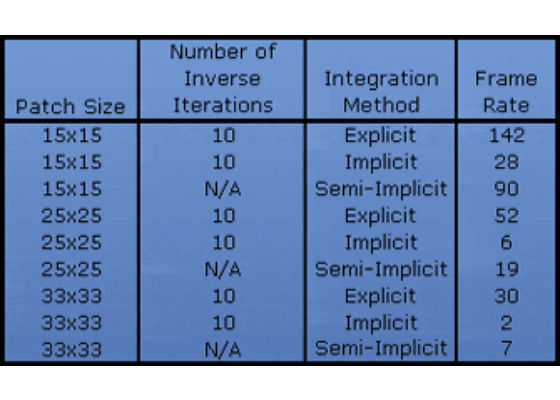Figure 5 - Performance results for various cloth sizes

Some interesting things to note about the performance that isn't shown in the figure are:

• Initialization time for the implicit method can be fairly large as the sparse matrices are allocated.
• Initialization time for the semi-implicit method can be considerably larger than that for the implicit method because a large matrix (1089x1089 in the 33x33 patch case) needs to be inverted. The same amount of computation would be required any time the time step changed.
• The implicit method is the only one that uses the actual spring strengths to hold the cloth together. Because of this, it may be necessary to increase the spring constants when using the implicit method.
• Desbrun claimed being able to vary the strength of the spring constant by a factor of 106 without causing instability. I was only able to achieve a factor of 105 which makes me think that other simulation specifics (like particle masses) may have been different.
• For the explicit and semi-implicit cases I needed to make the mass of the particles fairly large to achieve stability with a time step of 0.02 seconds. This could cause the cloth to have unusual properties if incorporated with other physics simulation involving inertia and collisions. In your game you may want to maintain separate masses for the updating of the cloth and the interaction of the cloth with the world.
• Because I haven't implemented real collision detection it's uncertain how collision with other objects will affect the stability and hence the performance of the various implementations.
• I maintained a linked list of spring forces that needed to be applied and then have their deformation constraints applied. Performance could be improved by storing these in an array that could be more quickly walked through.

Even though explicit integration seems to work best for my test case, the benefits of implicit integration should not be overlooked. Implicit integration can stably handle extremely large forces without blowing up. Explicit integration schemes cannot make such a claim. And while deformation constraints can be used with explicit integration to provide realistic looking cloth, implicit integration would have to be used if a more physically accurate simulation of cloth was required.

Conclusion

I breezed through some of the math and background with the hope that the accompanying source code would be even more valuable than a theoretical explanation which can be found in other more academic papers. Feel fr ee to take parts of the code and incorporate it in your title. There's a lot more that can be done than what I've presented here. Start simple and add a wind force and remember that it should affect triangles created by the particles not the particles themselves. Or try adding a user controllable mouse force to drag the cloth around. Depending on whether you want to use cloth simulation for eye candy in your game (like flags blowing in the wind or the sail on a ship) or as a key element, you'll probably need collision detection at some point. Keep in mind that cloth-cloth collision detection can be difficult to do efficiently.

Well, I've taken a brief look at real-time simulation of realistic looking cloth and hopefully have presented something of use to you in your game development. I look forward to seeing new games that incorporate various aspects of physics simulation with cloth simulation as one of them.

For more such intel resources and tools from Intel on Game, please visit the Intel® Game Developer Zone

Source:https://software.intel.com/en-us/articles/simulating-cloth-for-3d-gamesPromotion